# scipy.spatial.distance.sokalsneath¶

scipy.spatial.distance.sokalsneath(u, v)

Computes the Sokal-Sneath dissimilarity between two boolean vectors u and v,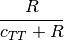where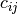is the number of occurrences of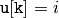and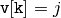for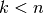and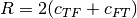.

Parameters : u : ndarray An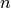-dimensional vector. v : ndarray An-dimensional vector. d : double The Sokal-Sneath dissimilarity between vectors u and v.

#### Previous topic

scipy.spatial.distance.sokalmichener

#### Next topic

scipy.spatial.distance.sqeuclidean